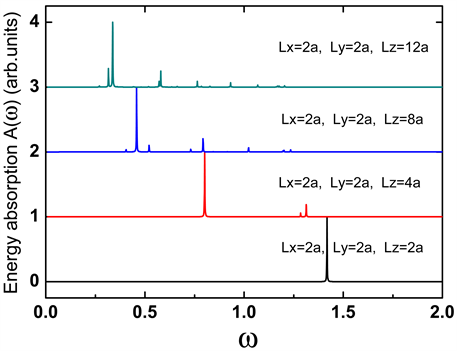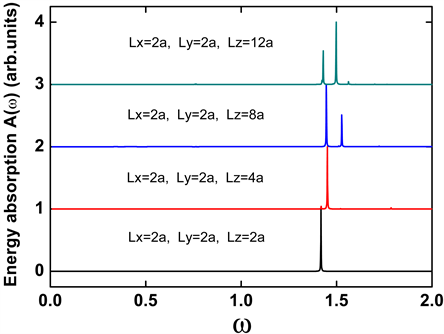方柱形原子团簇中的纵向等离激元和横向等离激元Longitudinal Plasmon and Transverse Plasmon in Square Cylinder

• 全文下载: PDF(783KB)    PP.259-263   DOI: 10.12677/MP.2018.85028
• 下载量: 287  浏览量: 678   科研立项经费支持

Based on the linear response theory in the random-phase approximation and the free-electron gas model, we study the plasmon excitations in square cylinder. Results show: when the length of square cylinder is increased, the frequency of longitudinal plasmon displays redshift, but the frequency of transverse plasmon shows blue shift.

1. 引言

Nie发现，Ag纳米颗粒上等离激元激发可使单分子拉曼散射信号的增强因子高达1014，是探测和分析单分子特性的有效工具  。与单个颗粒的增强效果相比，一对金属纳米颗粒构成的光学天线在等离激元共振激发时会引起电荷的重新分布，并在其缝隙处发生近场耦合，使得该处电场引起的双光子信号增强80倍以上，这为在纳米尺度上调控物质的光物理过程提供了新手段和新技术  。等离激元产生的非线性光学增强现象可以调控自身在微纳结构中的传播效应。例如，结合等离激元对环境折射率敏感的特性，以及非线性介质的光克尔效应(介质的折射率随光强发生变化)，利用控制光场改变非线性介质的折射率，从而改变等离激元的传输特性，这一现象可用于制备亚波长光学电路中的光开关  。

2. 研究方法

${\psi }_{{n}_{x}{n}_{y}{n}_{z}}\left(x,y,z\right)=C\mathrm{sin}\frac{{n}_{x}\text{π}x}{{L}_{x}a}\mathrm{sin}\frac{{n}_{y}\text{π}y}{{L}_{y}a}\mathrm{sin}\frac{{n}_{z}\text{π}z}{{L}_{z}a}$ (1)

${E}_{{n}_{x}{n}_{y}{n}_{z}}=\frac{{\text{π}}^{2}{\hslash }^{2}}{2{m}_{e}{a}^{2}}\left(\frac{{n}_{x}^{2}}{{L}_{x}^{2}}+\frac{{n}_{y}^{2}}{{L}_{y}^{2}}+\frac{{n}_{z}^{2}}{{L}_{z}^{2}}\right)$ (2)

${\rho }^{ind}\left(r,\omega \right)=\int \prod \left(r,{r}^{\prime },\omega \right)V\left({r}^{\prime },\omega \right)\text{d}{r}^{\prime }$ (3)

$\prod \left(r,{r}^{\prime },\omega \right)=2{e}^{2}\underset{nm}{\sum }\frac{f\left({E}_{n}\right)-f\left({E}_{m}\right)}{{E}_{n}-{E}_{m}-\omega -i\gamma }{\psi }_{n}^{\ast }\left(r\right){\psi }_{m}\left(r\right){\psi }_{m}^{\ast }\left({r}^{\prime }\right){\psi }_{n}\left({r}^{\prime }\right)$ (4)

${V}^{ind}\left(r,\omega \right)=\frac{1}{4\text{π}{\epsilon }_{0}}\int \frac{{\rho }^{ind}\left({r}^{\prime },\omega \right)}{|r-{r}^{\prime }|}\text{d}{r}^{\prime }$ (5)

${V}^{ind}\left(r,\omega \right)=2{e}^{2}\underset{nm}{\sum }\frac{f\left({E}_{n}\right)-f\left({E}_{m}\right)}{{E}_{n}-{E}_{m}-\omega -i\gamma }×\frac{1}{4\text{π}{\epsilon }_{0}}\int \frac{{\psi }_{n}^{\ast }\left({r}^{\prime }\right){\psi }_{m}\left({r}^{\prime }\right)}{|r-{r}^{\prime }|}\text{d}{r}^{\prime }\left[{V}_{nm}^{ext}\left(\omega \right)+{V}_{nm}^{ind}\left(\omega \right)\right]$ (6)

${V}_{nm}\left(\omega \right)=\int {\psi }_{n}^{\ast }\left(r\right)V\left(r,\omega \right){\psi }_{m}\left(r\right)$ (7)

${V}_{{n}^{\prime }{m}^{\prime }}^{ind}\left(\omega \right)=2{e}^{2}\underset{nm}{\sum }\frac{f\left({E}_{n}\right)-f\left({E}_{m}\right)}{{E}_{n}-{E}_{m}-\omega -i\gamma }×\frac{1}{4\text{π}{\epsilon }_{0}}\int \frac{{\psi }_{n}^{\ast }\left({r}^{\prime }\right){\psi }_{m}\left({r}^{\prime }\right){\psi }_{{n}^{\prime }}^{\ast }\left(r\right){\psi }_{{m}^{\prime }}\left(r\right)}{|r-{r}^{\prime }|}\left[{V}_{nm}^{ext}\left(\omega \right)+{V}_{nm}^{ind}\left(\omega \right)\right]\text{d}{r}^{\prime }\text{d}r$ (8)

${M}_{{n}^{\prime }{m}^{\prime },nm}\left(\omega \right)=\frac{2{e}^{2}}{4\text{π}{\epsilon }_{0}}\underset{nm}{\sum }\frac{f\left({E}_{n}\right)-f\left({E}_{m}\right)}{{E}_{n}-{E}_{m}-\omega -i\gamma }\int \frac{{\psi }_{{n}^{\prime }}^{\ast }\left(r\right){\psi }_{{m}^{\prime }}\left(r\right){\psi }_{n}^{\ast }\left({r}^{\prime }\right){\psi }_{m}\left({r}^{\prime }\right)}{|r-{r}^{\prime }|}\text{d}{r}^{\prime }\text{d}r$ (9)

$\underset{nm}{\sum }\left[{\delta }_{{n}^{\prime }{m}^{\prime },nm}-{M}_{{n}^{\prime }{m}^{\prime },nm}\left(\omega \right)\right]{V}_{nm}^{ind}\left(\omega \right)=\underset{nm}{\sum }{M}_{{n}^{\prime }{m}^{\prime },nm}\left(\omega \right){V}_{nm}^{ext}\left(\omega \right)$ (10)

$A\left(\omega \right)=-\frac{1}{2}\omega \mathrm{Im}\left\{\int {\rho }^{ind}\left(r,\omega \right){\left[{V}^{ext}\left(r,\omega \right)\right]}^{\ast }\right\}$ (11)

3. 结果与分析

4. 结论Figure 1. The evolutions of longitudinal plasmon with the length of square cylinderFigure 2. The evolutions of longitudinal plasmon with the length of square cylinder

NOTES

*通讯作者。

  Ebbesen, T.W., Lezec, H.J. and Ghaemi, H.F. (1988) Extraordinary Optical Transmission through Sub-Wavelength Hole Arrays. Nature, 391, 667-66. https://doi.org/10.1038/35570  Thio, T., Pellerin, K.M. and Linke, R.A. (2001) Enhanced Light Transmission through a Single Subwavelength Aperture. Optics Letters, 26, 1972-1974. https://doi.org/10.1364/OL.26.001972  McFarland, A.D. and Van Duyne, R.P. (2003) Single Silver Nanoparticles as Real-Time Optical Sensors with Zeptomole Sensitivity. Nano Letters, 3, 1057-1062. https://doi.org/10.1021/nl034372s  Brolo, A.G., Gordon, R. and Leathem, B. (2004) Surface Plasmon Sensor Based on the Enhanced Light Transmission through Arrays of Nanoholes in Gold Films. Langmuir, 20, 4813-4815. https://doi.org/10.1021/la0493621  Wei, H., Hao, F. and Huang, Y. (2008) Polarization Dependence of Surface-Enhanced Raman Scattering in Gold Nanoparticle-Nanowire Systems. Nano Letters, 8, 2497-2502. https://doi.org/10.1021/nl8015297  Thyagarajan, K., Butet, J. and Martin, O.J.F. (2013) Augmenting Second Harmonic Generation Using Fano Resonances in Plasmonic Systems. Nano Letters, 13, 1847-1851. https://doi.org/10.1021/nl400636z  Lezec, H.J., Dionne, J.A. and Atwater, H.A. (2007) Negative Refraction at Visible Fre-quencies. Science, 316, 430-432. https://doi.org/10.1126/science.1139266  Huang, H., Wang, B. and Long, H. (2014) Plasmon-Negative Refraction at the Heterointerface of Graphene Sheet Arrays. Optics Letters, 39, 5957-5960. https://doi.org/10.1364/OL.39.005957  王振林. 表面等离激元研究新进展[J]. 物理学进展, 2009, 29(3): 287-324.  龚健, 张利伟, 陈亮, 等. 石墨烯基双曲色散特异材料的负折射与体等离子体性质[J]. 物理学报, 2015, 64(6): 067301.  Nie, S. and Emory, S.R. (1997) Probing Single Molecules and Single Nanoparticles by Surface-Enhanced Raman Scattering. Science, 275, 1102-1106. https://doi.org/10.1126/science.275.5303.1102  Ghenuche, P., Cherukulappurath, S. and Taminiau, T.H. (2008) Spectros-copic Mode Mapping of Resonant Plasmon Nanoantennas. Physical Review Letters, 101, 116805. https://doi.org/10.1103/PhysRevLett.101.116805  Wurtz, G.A., Pollard, R. and Zayats, A.V. (2006) Optical Bistability in Nonlinear Surface-Plasmon Polaritonic Crystals. Physical Review Letters, 97, 057402. https://doi.org/10.1103/PhysRevLett.97.057402  Xue, H.J., Hao, D.P., Zhang, M. and Wang, X.M. (2017) Plasmon Excita-tions in the Dimers Formed by Atom Chains. Physica E, 86, 292-296. https://doi.org/10.1016/j.physe.2016.10.030  Xue, H.J., Wu, R.L. and Hu, C.X. (2018) The Study of the Plasmon Modes of Square Atomic Clusters Based on the Eigen-Oscillation Equation of Charge Under the Free-electron Gas Model. International Journal of Modern Physics B, 32, 1850139. https://doi.org/10.1142/S0217979218501394